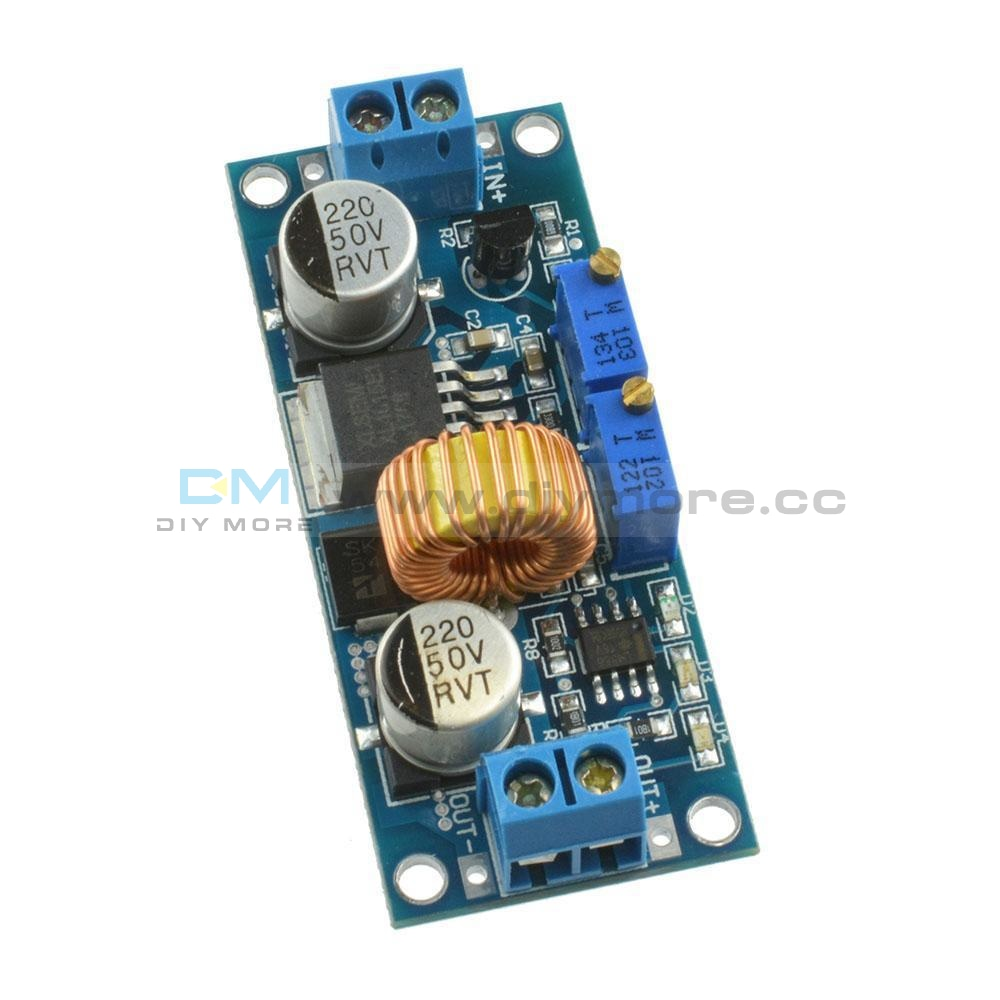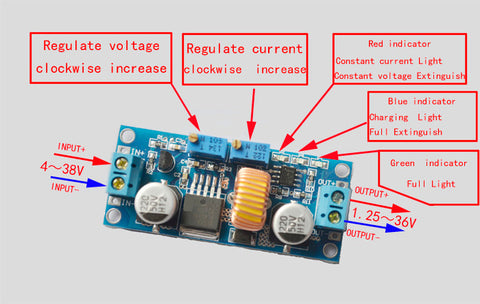### 5A CV CC Step Down Lithium Charger Buck LED Driver Power Supply Module### 5A CV CC Step Down Lithium Charger Buck LED Driver Power Supply Module

SKU:010515
Regular price \$4.99Specifications:
Input voltage range:6～38VDC(Note:input voltage not exceeding 38V)
Output current: 0-5A; Output power: 75W
High efficiency up to 96%
Built in thermal shutdown function, current limit function and output short protection function.
Input reverse polarity protection: None (if required, high current diode in series with the input).

Size : 61.7*26.2*15mm
Weight: 20g

Application
1、Use as a step-down modules with overcurrent protection capabilities
Usage:
(1) adjust the “voltage potentiometer” so that the output voltage reaches the value you want.
(2) use a multimeter to measure the output short-circuit current with 10A stalls, while regulating the “current potentiometer” so that the output current reaches a predetermined overcurrent protection value. (For example, the meter displays the current value of 4A, then you can use the module to a maximum current of 4A)

2、Use as a battery charger
Usage:
(1). Make sure you need to charge the battery float voltage and charging current; (if lithium parameters 3.7V/2200mAh, then the float voltage is 4.2V, the maximum charging current 1C, ie 2200mA)
(2). Under no-load conditions, adjust the “Voltage potentiometer” so that the output voltage reaches the float voltage; (if to 3.7V rechargeable lithium battery, the output voltage can be adjusted to 4.2V)
(3). use a multimeter to measure the output short-circuit current with 10A stalls, while regulating the “current potentiometer” so that the output current reaches a predetermined Charging current value.
(4). Charge turn lamp current factory default is 0.1 times the charging current; (Battery during charging current is gradually reduced, if the charge current setting is 1A, then when the charge current is less than 0.1A, blue lights turned off, the green light is on, which means that the battery is fully charged)
(5).connected to the battery charge.
(1,2,3,4 steps as: Output is unloaded, do not connect the battery)

3、Use as a LED constant current driver module
Usage:
(1). adjust the “voltage potentiometer” so that the output voltage reaches the value you want.
(2). use a multimeter to measure the output short-circuit current with 10A stalls, while regulating the “current potentiometer” so that the output current reaches a predetermined LED operating current.
(3). Connect LED, work.
(1,2 steps as: Output is unloaded, do not connect LED)Package included:

1 x  5A Lithium Charger CV CC buck Step down Power Supply Module LED Driver• guaranteeQuality checked
• Free return Within 60 days
• Consultancy86-0755-85201155

Specifications:
Input voltage range:6～38VDC(Note:input voltage not exceeding 38V)
Output current: 0-5A; Output power: 75W
High efficiency up to 96%
Built in thermal shutdown function, current limit function and output short protection function.
Input reverse polarity protection: None (if required, high current diode in series with the input).

Size : 61.7*26.2*15mm
Weight: 20g

Application
1、Use as a step-down modules with overcurrent protection capabilities
Usage:
(1) adjust the “voltage potentiometer” so that the output voltage reaches the value you want.
(2) use a multimeter to measure the output short-circuit current with 10A stalls, while regulating the “current potentiometer” so that the output current reaches a predetermined overcurrent protection value. (For example, the meter displays the current value of 4A, then you can use the module to a maximum current of 4A)

2、Use as a battery charger
Usage:
(1). Make sure you need to charge the battery float voltage and charging current; (if lithium parameters 3.7V/2200mAh, then the float voltage is 4.2V, the maximum charging current 1C, ie 2200mA)
(2). Under no-load conditions, adjust the “Voltage potentiometer” so that the output voltage reaches the float voltage; (if to 3.7V rechargeable lithium battery, the output voltage can be adjusted to 4.2V)
(3). use a multimeter to measure the output short-circuit current with 10A stalls, while regulating the “current potentiometer” so that the output current reaches a predetermined Charging current value.
(4). Charge turn lamp current factory default is 0.1 times the charging current; (Battery during charging current is gradually reduced, if the charge current setting is 1A, then when the charge current is less than 0.1A, blue lights turned off, the green light is on, which means that the battery is fully charged)
(5).connected to the battery charge.
(1,2,3,4 steps as: Output is unloaded, do not connect the battery)

3、Use as a LED constant current driver module
Usage:
(1). adjust the “voltage potentiometer” so that the output voltage reaches the value you want.
(2). use a multimeter to measure the output short-circuit current with 10A stalls, while regulating the “current potentiometer” so that the output current reaches a predetermined LED operating current.
(3). Connect LED, work.
(1,2 steps as: Output is unloaded, do not connect LED)Package included:

1 x  5A Lithium Charger CV CC buck Step down Power Supply Module LED Driver